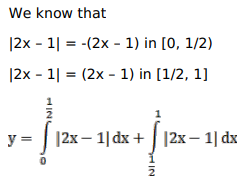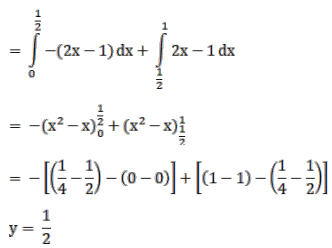# Solve this following

Question:

Mark $(\sqrt{)}$ against the correct answer in the following:

$\int_{0}^{1}|2 x-1| d x=?$

A. 2

B. $\frac{1}{2}$

C. 1

D. 0

Solution: